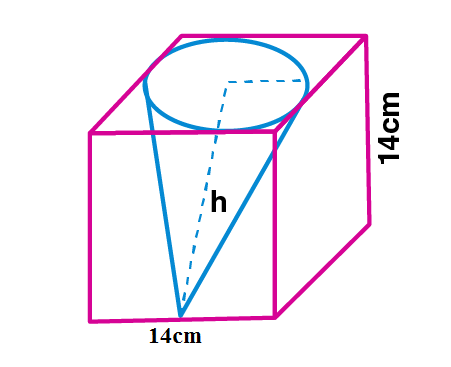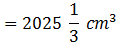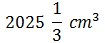Newbie

# From a cube of edge 14 cm, a cone of maximum size is carved out. Find the volume of the remaining material.

• 1

This is the Important question of class 10 Based on Mensuration Chapter of M.L Aggarwal book for ICSE BOARD.
Here the edge cube is given , a cone of maximum size is carved out then find the volume of the remaining material.
This is the Question Number 03, Exercise 17.4 of M.L Aggarwal.

Share

1.Given edge of the cube, a = 14 cm

Radius of the cone, r = 14/2 = 7 cm

Height of the cone, h = 14 cm

Volume of the cube = a3

= 143

= 14×14×14

= 2744 cm3

Volume of the cone = (1/3)r2h

= (1/3)×(22/7)×72×14

= 22×7×14/3

= 2156/3 cm3

Volume of the remaining material = Volume of the cube- Volume of the cone

= 2744-2156/3

= (3×2744-2156)/3

= (8232 – 2156)/3

= 6076/3Hence the volume of the remaining material is.

• 0